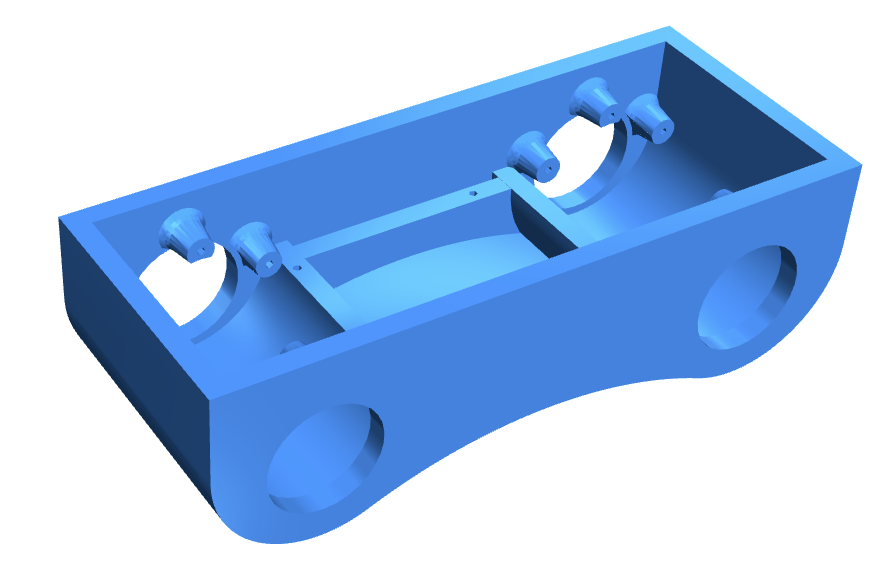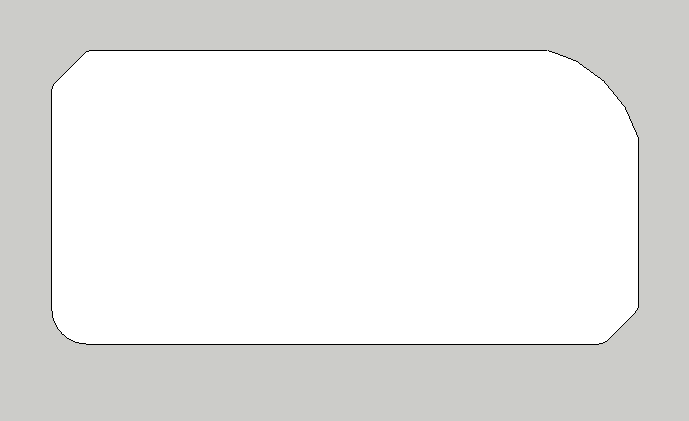# Shape generator

• While working on the design below in OpenSCAD, I realized that Boolean construction of a shape can become a bit counter intuitive when you start working with curves. The bottom of this box curves up but the curve is elliptical as well as the curves on either side. All curves are tangential to each other.In order to construct shapes easily, I have been looking for a code based way to generate a shape outline. For that I have been taking queues from the SVG path specifications where you start at a given point and a sequence of instructions defines the path. I like that those commands can use either relative or absolute coordinates depending on the command.

However, I also want easy ways to fillet or chamfer corners, tangent align an arc to another line or arc and so on.

Some thoughts:

• Shape generator gives me a points list or a polygon.
• All points to be calculated without the use of boolean construction.
• Commands need to be high level. A render step is required at the end.
• Didn't think about constraints much but edges could be named and referenced.

Consider this shape:The code could look like this compliant with JSCAD Conventions... which states function parameters should be passed as a JSON object.

``````var boxWidth = 50;
var boxLength = 100;
var boxHeight = 40;
var boxCut = 15;
var boxRadius = 30;

//  Sets start point and global fillet parameter for all edge joins
Shape.init({point:[3,4], fillet:2});

//  move to point relative from the init point and fillet that corner with given value
Shape.move({point:[-boxWidth/2,-boxHeight/2], fillet:6});

//  draws vertically along y axis by given distance and chamfer that corner with given value
Shape.vert({dist:boxWidth, chamfer:6});

//  draws horizontally along y axis by given distance
Shape.horz({dist:boxLength - 15});

//  draws an arc tangential to the previous edge to given point with given radius. Other parameters can modify that behavior.
Shape.arc ({point:[15,15], radius: boxRadius});

//  draws vertically along y axis by given distance and chamfer that corner with given value
Shape.vert({d:-boxWidth + 15});

var boxPolygon = polygon(Shape.points);
//or
var boxPolygon = Shape.polygon;
``````

The "JSCAD Conventions..." cause us to loose Autocomplete and code suggestion in our editors though. But as we use JSON notation we might as well do the whole thing like that.

``````      var boxWidth = 50;
var boxLength = 100;
var boxHeight = 40;
var boxCut = 15;
var boxRadius = 30;

Shape.steps = [
{ c: 'start', point:[0,0], fillet:2 },
{ c: 'move', point:[-boxWidth/2,-boxHeight/2], fillet:6 },
{ c: 'vert', dist:boxWidth, chamfer:6 },
{ c: 'horz', dist:boxLength - boxCut },
{ c: 'arc', point:[boxCut,-boxCut], r: boxRadius },
{ c: 'vert', dist: -boxWidth + boxCut}
]

var boxPolygon = polygon(Shape.points);

//or

var boxPolygon = Shape.polygon;
``````

Of course now you loose even more of the editors autocomplete logic. But it's clean and very versatile.

Essentially the command list represents the edges of the shape. From here we can even start to look at edge fillet etc.

Note: A fillet cuts into the corner while an arc starts and ends at a precise coordinate.

The shape generator should also have turtle like behavior such as

``````Shape.turn({angle: 20});   //  turns relative to the current direction
Shape.Turn({angle:20});    //  sets current direction to angle value
Shape.forward({dist:25});  //  draws line in current direction

//Capitalization of a command determines relative or absolute behavior where sensible.
``````

Not even sure if code like this should be an external library of incorporated in JSCAD.
I love to hear what you guys think of the whole shape generator thing.

• I have had similar thoughts about getting a drawing tool like this into jscad, I believe it could not only be useful for extruding simple shapes, but also, if constraints are possible, for the layout of all sorts of things.

This project looks interesting https://github.com/IjzerenHein/kiwi.js .. though I admit I dont quite understand how you would get anything complicated into the calculations.

• @Dinther Excellent! I didn’t know about constraints as well. Interesting...

But the example is horrid to read, and even worse to understand.Maybe that’s why SVG has adopted a more simple approach to defining paths. Simple is best and even better if it actually makes sense to ordinary people.

Having said that... the underlying implementation could use a constraints based object, and there could be a simple API above that. For example, users don’t use mat4 (matrix) but the transforms create matrices for all operations on geometry.

• I had never heard of maker.js That is an impressive piece of kit although I found it very hard to read the code for their sample models. Pulled a bunch of good ideas from it though.

In my initial post I had not considered constraints.

That might be a mistake. I picked up FreeCAD again and spend some time learning to use a constraint system. Blame it to years of using grid systems but only now the penny drops that with constraints you have no need for coordinates!

Looked around for some javascript based solvers and only found https://github.com/tmpvar/2d-constraints-bfgs which lacks constraints such as tangent and it's rather old. Another promising but not complete solver is https://github.com/tab58/assemble2d. But let's assume this get's solved (pun intended).

Pseudo code for the same example shape could be:

``````0    var shapePoints = Chain.clear().
1      edge().vert().length(boxWidth).fillet(2).chamfer(6).fillet(2).
2      edge().horz().length(boxLength - boxCut).fillet(2).
3      edge().radius(boxRadius).out().distH(boxCut).distV(-boxCut]).fillet(2).
4      edge().vert().length(-boxWidth + boxCut).fillet(2).
5      edge().fillet(6).points;
``````

In the above code the shape is build up out of edges going clockwise which are straight by default and the then following constraints apply to that edge with at the end corner modifiers. This way each function has only zero or one parameter. The assumption is made that the end point of each edge coincides with the start point of the next edge.

Not sure yet how the syntax would look like to position the shape relative to the origin point as it requires constraints to multiple arbitrary points in the shape.

• Really nice start. Thanks, @Dinther

One of the best libraries in this space is Makerjs by @danmarshall

You could take some hints from here.